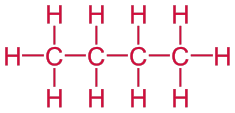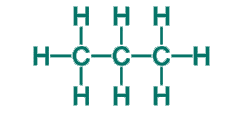# Structural Formula

All-round us we have Chemical compounds – they are in our beverages, food and in items, one uses in the day to day. We can identify these chemical compounds by their molecular formulas. Though the molecular formula demonstrates how many atoms there are for every element existing in the compound, it does not communicate to us about by what means the elements are attached to each other. We have no idea which atom of an element is attached to which. This is the place where the structural formula arises. It displays how the atoms are organized and attached together in a molecular formula of a chemical compound. Deprived of the structural formula, one would not discern that acetic acid has a carbon atom double-bonded together with oxygen. One can see the spatial arrangement of the atoms in the compound, by taking a look at the formula.

The electron dot structural formula depiction uses dots to signify the electrons involved with the bonding amid different atoms. The line-bond structural formula is a very frequently used depiction of the structural formula. As the term puts forward, the line-bond structural formula makes use of lines and bonds to show the covalent bonds between atoms.

The condensed formula still makes use of lines amongst bonded atoms but is a stress-free and smaller method to draw the line-bond structural formula because it omits the carbon and hydrogen bonds.

## Examples

Problem 1: Draw the structural formula of butane. It is a linear alkane that contains four carbon atoms is called butane.

Solution: Begin by drawing a skeleton that contains four carbon atoms.

C — C — C — C

Then add enough bonds so that each carbon atom forms a total of four bonds and add hydrogen atoms to complete the structure.Problem 2: Draw the condensed structural formula of propane.

Solution: List each carbon atom in the molecule and then add the hydrogen atoms that are bonded to each carbon.